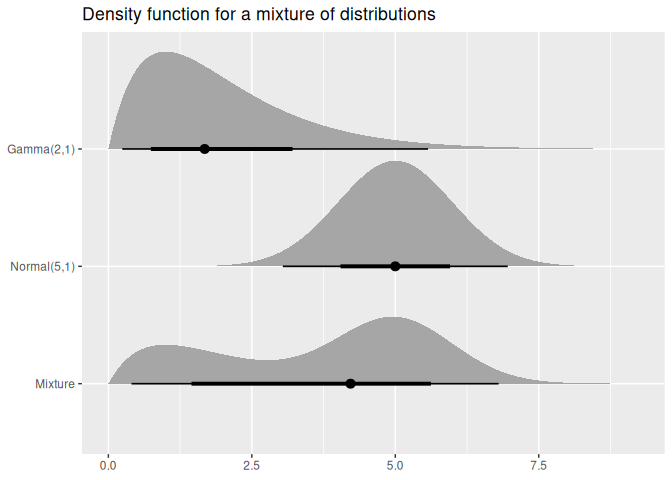The distributional package allows distributions to be used in a vectorised context. It provides methods which are minimal wrappers to the standard d, p, q, and r distribution functions which are applied to each distribution in the vector. Additional distributional statistics can be computed, including the mean(), median(), variance(), and intervals with hilo().

The distributional nature of a model’s predictions is often understated, with defaults of predict() methods usually only producing point predictions. The forecast() function from the forecast package goes further in illustrating uncertainty by producing point forecasts and intervals by default, however the user’s ability to interact with them is limited. This package vectorises distributions and provides methods for working with them, making entire distributions suitable prediction outputs for model functions.

## Installation

You can install the released version of distributional from CRAN with:

install.packages("distributional")

The development version can be installed from GitHub with:

# install.packages("remotes")
remotes::install_github("mitchelloharawild/distributional")

## Examples

Distributions are created using dist_*() functions. Currently only the normal distribution is supported for testing purposes.

library(distributional)
#>
#> Attaching package: 'distributional'
#> The following object is masked from 'package:grDevices':
#>
#>     pdf
my_dist <- c(dist_normal(mu = 0, sigma = 1), dist_student_t(df = 10))
my_dist
#> <distribution>
#>  N(0, 1)     t(10, 0, 1)

The standard four distribution functions in R are usable via these generics:

density(my_dist, 0) # c(dnorm(0, mean = 0, sd = 1), dt(0, df = 10))
#>  0.3989423 0.3891084
cdf(my_dist, 5) # c(pnorm(5, mean = 0, sd = 1), pt(5, df = 10))
#>  0.9999997 0.9997313
quantile(my_dist, 0.1) # c(qnorm(0.1, mean = 0, sd = 1), qt(0.1, df = 10))
#>  -1.281552 -1.372184
generate(my_dist, 10) # list(rnorm(10, mean = 0, sd = 1), rt(10, df = 10))
#> []
#>    1.262954285 -0.326233361  1.329799263  1.272429321  0.414641434
#>   -1.539950042 -0.928567035 -0.294720447 -0.005767173  2.404653389
#>
#> []
#>    0.99165484 -1.36999677 -0.40943004 -0.85261144 -1.37728388  0.81020460
#>   -1.82965813 -0.06142032 -1.33933588 -0.28491414

You can also compute intervals using hilo()

hilo(my_dist, 0.95)
#> <hilo>
#>  [-0.01190677, 0.01190677]0.95 [-0.01220773, 0.01220773]0.95

Additionally, some distributions may support other methods such as mathematical operations and summary measures. If the methods aren’t supported, a transformed distribution will be created.

my_dist
#> <distribution>
#>  N(0, 1)     t(10, 0, 1)
my_dist*3 + 2
#> <distribution>
#>  N(2, 9)        t(t(10, 0, 1))
mean(my_dist)
#>  0 0
variance(my_dist)
#>  1.00 1.25

You can also visualise the distribution(s) using the ggdist package.

library(ggdist)
library(ggplot2)

df <- data.frame(
name = c("Gamma(2,1)", "Normal(5,1)", "Mixture"),
dist = c(dist_gamma(2,1), dist_normal(5,1),
dist_mixture(dist_gamma(2,1), dist_normal(5, 1), weights = c(0.4, 0.6)))
)

ggplot(df, aes(y = factor(name, levels = rev(name)))) +
stat_dist_halfeye(aes(dist = dist)) +
labs(title = "Density function for a mixture of distributions", y = NULL, x = NULL)